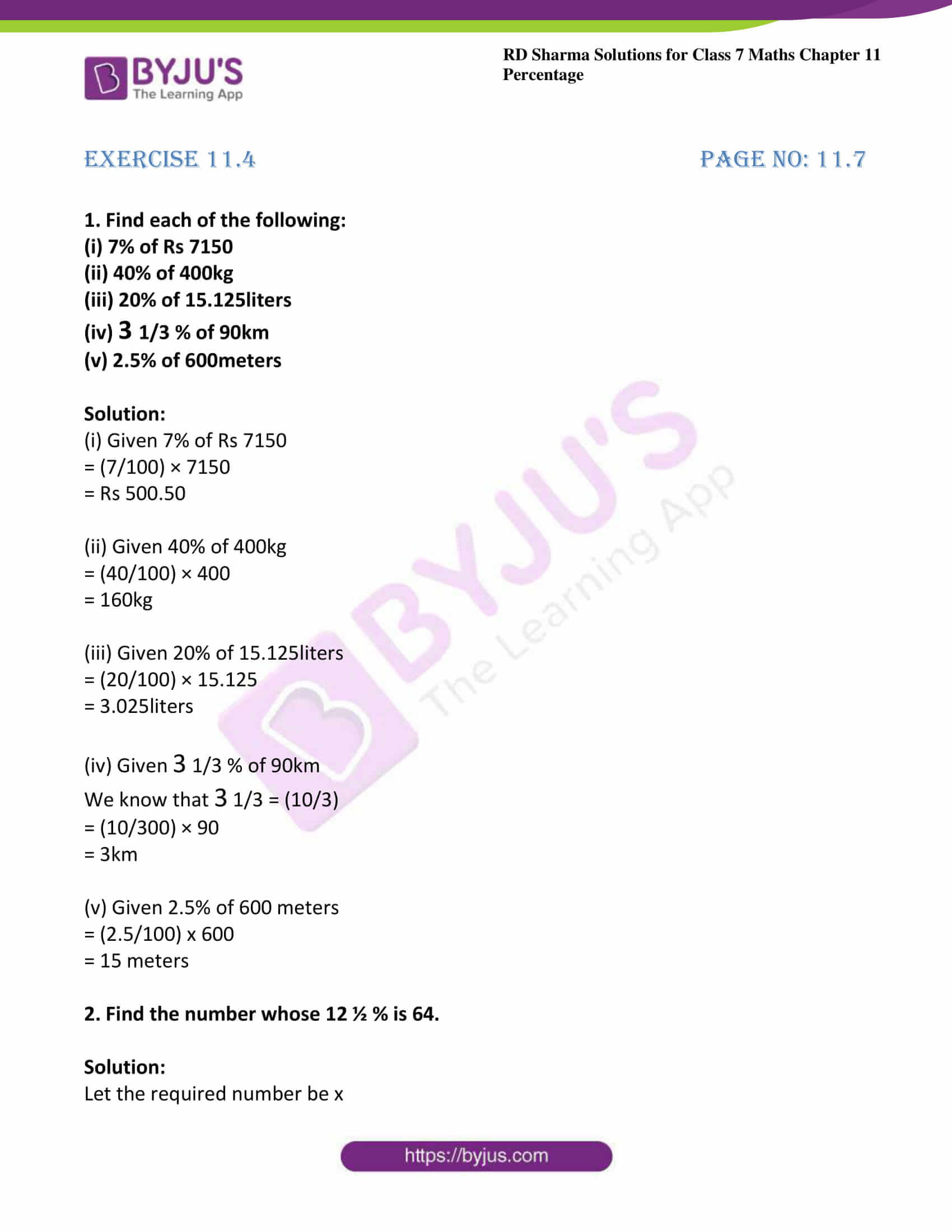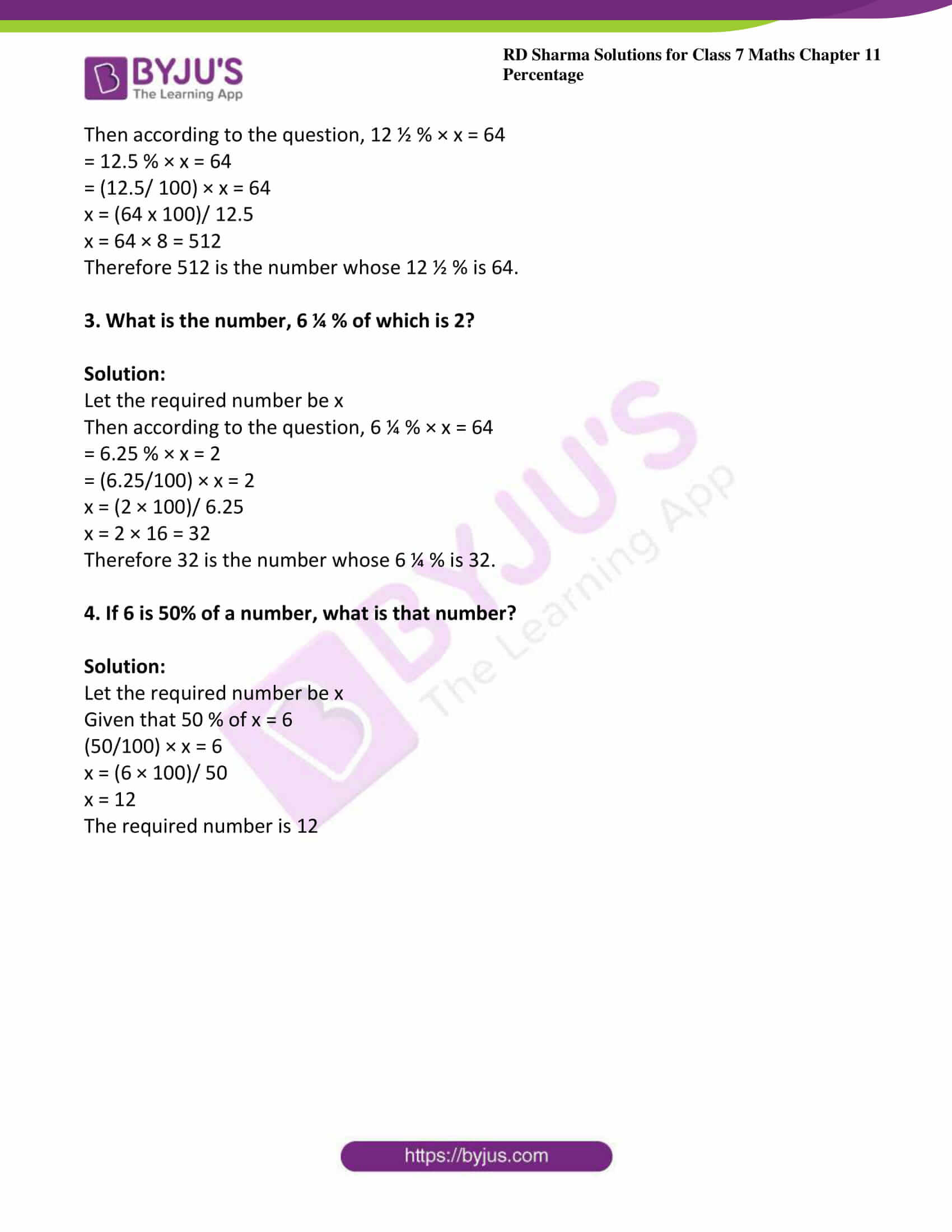# RD Sharma Solutions For Class 7 Maths Chapter 11 Percentage Exercise 11.4

The PDF of RD Sharma Solutions for Class 7 Exercise 11.4 are available here. The solutions for exercise wise problems are prepared by BYJU’S experts in Maths after conducting vast research on each topic. The step-wise solutions prepared by subject experts help students to solve problems with competence. By practising RD Sharma Solutions for Class 7, students will be able to solve all questions present in them without any difficulties. This exercise contains problems on finding the percentage of a given number. By practising previous exercises, students become thorough about percent but here they learn about the calculation of percentage.

## Download the PDF of RD Sharma Solutions For Class 7 Maths Chapter 11 – Percentage Exercise 11.4### Access answers to Maths RD Sharma Solutions For Class 7 Chapter 11 – Percentage Exercise 11.4

Exercise 11.4 Page No: 11.7

1. Find each of the following:

(i) 7% of Rs 7150

(ii) 40% of 400kg

(iii) 20% of 15.125liters

(iv) 3 1/3 % of 90km

(v) 2.5% of 600meters

Solution:

(i) Given 7% of Rs 7150

= (7/100) × 7150

= Rs 500.50

(ii) Given 40% of 400kg

= (40/100) × 400

= 160kg

(iii) Given 20% of 15.125liters

= (20/100) × 15.125

= 3.025liters

(iv) Given 3 1/3 % of 90km

We know that 3 1/3 = (10/3)

= (10/300) × 90

= 3km

(v) Given 2.5% of 600 meters

= (2.5/100) x 600

= 15 meters

2. Find the number whose 12 ½ % is 64.

Solution:

Let the required number be x

Then according to the question, 12 ½ % × x = 64

= 12.5 % × x = 64

= (12.5/ 100) × x = 64

x = (64 x 100)/ 12.5

x = 64 × 8 = 512

Therefore 512 is the number whose 12 ½ % is 64.

3. What is the number, 6 ¼ % of which is 2?

Solution:

Let the required number be x

Then according to the question, 6 ¼ % × x = 64

= 6.25 % × x = 2

= (6.25/100) × x = 2

x = (2 × 100)/ 6.25

x = 2 × 16 = 32

Therefore 32 is the number whose 6 ¼ % is 32.

4. If 6 is 50% of a number, what is that number?

Solution:

Let the required number be x

Given that 50 % of x = 6

(50/100) × x = 6

x = (6 × 100)/ 50

x = 12

The required number is 12